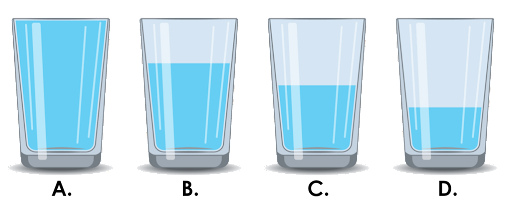# What Is Quantity? Definition with Examples

## What Is a Quantity in Math?

Quantity describes an amount or a number of something. It is the measurement of something by saying how much of it there is. It is an aspect or property of an object which can be measured.

A quantity is a measurable property of an object. Usually, it is the product of a number (magnitude) and a unit, e.g. 35 lb.

We talk about quantity in terms of an amount or the total number of items or simply the size or extent of something that is measurable. It answers the questions like “how much” or “how many” of something is present. We can compare quantities as “more,” “less,” or “equal.”

The abbreviation for quantity is “qty.”

Examples: volume, mass, length, people, number, (x + y), area, etc

We refer to quantity in many day-to-day situations.

• The quantity of milk required is mentioned in the recipe.
• Is the quantity of food sufficient for the party?
• The quantity of oil used is too less.
• How much coffee did you have? A cup of coffee!
• How many eggs are in the box? A dozen eggs!

## Quantity in Math: Definition

In math, the definition of quantity can be given as anything that can be counted or measured. Quantity is a generic term used to express the measurement (count or amount). It is an amount that you can measure.

## Quantities in Algebra

What does quantity mean in algebra? An expression as one whole represents a quantity.

We can read (x + 9)2 as “x plus 9, the quantity squared.”

In a math equation, a quantity is any number or variable and any algebraic combination of other quantities. Thus, something on which some mathematical operation can be performed is also referred to as “quantity.”

In algebra, quantities are usually represented by variables, and they can be unknown or vary in value.

We can write expressions or equations involving an unknown as follows:

• five times the quantity = 4x
• the quantity of x plus three = x + 3

## Quantities in Geometry

The quality of something that makes it measurable is quantity. Example: length, area, volume, height, etc.

One important point to be remembered is that we cannot compare quantities that have different dimensions. For example, a square of side length of 2 inches will have an area of 4 inch2. Here, we cannot compare the side of 2 inches and the area of 4 inch2 as both quantities depict different aspects of the square.

Also, we cannot compare two quantities having different units. We first need to convert them into the same unit.

## Quantities in Physics

Physical quantities are properties of objects that can be quantified or anything that can be given a magnitude or a value. There are two types of physical quantities.

Base quantity: Base quantities are physical quantities that cannot be defined in terms of other base quantities. In simple words, they are independent quantities.

Examples: length, mass, time, temperature, etc.

Derived quantity: As the name suggests, derived quantities are “derived” from the base quantities. They are dependent on the base quantities.

Examples: Speed $= \frac{Distance}{Time}$ , Area, Volume

## Uses of Quantity

• Expressions of quantity show how many or how much of something there is. Quantity is used everywhere, in mathematics, science, and even in real life.
• We use quantity to define mathematical properties and relations using numbers or variables.
• In real life, we use the concept of quantity while counting the number of things you bought when you go shopping or when you are stating your age (Yes, your age is also a quantity!).
• In mathematics, we use quantities in equations. It can be used to describe a whole expression, unknown value in an equation, or individual terms.

## Quantity in Day-to-day Life

We use the concept of quantities in daily life without even realizing it. Whenever we express how much of something is present (in a container, in a box), we use the term “quantity.”

Let’s see a few examples of quantity in daily life.

Example 1: What is the quantity of sugar in the given packet?

It is 4 lb or 1.8 kg.

Example 2: What is the quantity of strawberries shown in the image?

We can express the quantity in two ways here.

We can say “13 strawberries.”

We can express it in terms of weight and say “1.2 lb.”

Example 3: What is the quantity of money shown in the image?

p = 64
3

### The ______ has half a glass of water.glass A
glass B
glass C
glass D
CorrectIncorrect
The glass C is half filled with water.

In a math equation, a quantity is any number or variable and any algebraic combination of other quantities. In the equation x + 6 = 10, there are four quantities represented: 6, 10, x, and the sum of x and 7, x + 7.

A unit is a standard for the measurement of physical quantities of the same kind. It described the magnitude of the quantity. Any physical quantity is expressed using a numerical value and a unit. Example: length = 35 feet

Magnitude is the numerical value of how much/many unit(s) of something is present in a system. It is a mathematical term that is used in concepts such as vectors and geometry to give them an amount of quantity.

Scalar quantity is defined as when a physical quantity has some magnitude but no direction.

Example: Mass, Distance

Vector quantities are quantities with both magnitude and direction.

Example: Speed

Quantity in math refers to the amount, number, or measure of something.